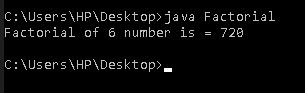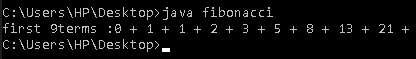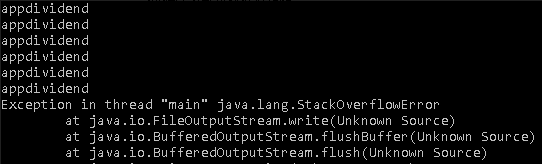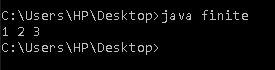# Recursion in Java Example | Java Recursion Tutorial

Recursion in Java is the process in which a method calls itself again and again, and the method that calls itself is known as the recursive method. In the real-time example, it’s like when you stand between two parallel mirrors and the image formed repeatedly. The method in Java that calls itself is called a recursive method. It makes the code compact, but complex to understand.

## Recursion in Java Example

In the recursive program, the solution to a base case is provided, and the solution to a bigger problem is expressed in terms of smaller problems.

See the following syntax.

```return_type method_name(argument-list)
{
//statements
method_name(argument-list);        /*calling the method continuously */
}
```

Let’s see some example of Recursion in java.

## #Factorial Program of a number

See the following program of factorial number in Java.

```class Factorial {
static int fact(int n) {
if (n == 1)
return 1;
else
return (n * fact(n - 1));
}

public static void main(String... args) {
System.out.println("Factorial of 6 number is = " + fact(6));
}
}```

See the following output.### #Explanation

In the above example, the fact function calls itself again and again. Like fact(6) calls fact(5), after that fact(5) calls fact(4) and so on till fact(1).

### #Execution process

 Fact(6)

 Fact(5)

 Fact(4)

 Fact(3)

 Fact(2)

 Fact(1)

return 1

return 2*1 =2

return 3*2*1 =6

return 4*3*2*1=24

return 5*4*3*2*1=120

return 6*5*4*3*2*1=720

## #Fibonacci series Program in Java

It is a series where the next term is the sum of the previous two terms. It starts with 0, 1 and so on.

See the following program.

```class fibonacci {
public static void main(String... args) {
int n = 9;
int a1 = 0, a2 = 1;
System.out.print("first " + n + "terms :");
for (int i = 1; i <= n; ++i) {
System.out.print(a1 + " + ");
int add = a1 + a2;
a1 = a2; /* swapping of number */
}
}
}```

See the following output.## #Infinite time recursion program in Java

In this program, the method is called infinite time. See the following code.

```class infinite {
static void v() {
System.out.println("appdividend");
v();
}

public static void main(String... args) {
v();
}
}```

See the following output.## #Finite time recursion

In this program, the method is called at a finite number of times like in factorial of a number and Fibonacci series.

```class finite {
public static void main(String... args) {
num(1);
}

static void num(int n) {
System.out.print(n + " ");
if (n == 3)
return;
num(n + 1);
}
}```

See the following output.## #Difference b/w Direct and Indirect recursion in Java

### Direct recursion

Direct recursion takes place when a method calls itself “directly” within its own body. Indirect recursion, no more than one method can be called by itself.

See the following code example.

```int sum( )
{
…
…
int sum( );
}
```

## #Indirect recursion

Indirect recursion takes place when a method calls another method, and that method calls the previous method back. Suppose a method A calls method B and method B calls again call method A, this is called indirect recursion. See the following example.

```int B( )
{
…
…
int A( );
}
int A( )
{
…
…
int B ( );
}
```

## #How a particular problem is solved using recursion

The idea is to represent the problem in terms of one or more smaller problems, and add one or more base conditions that stop the recursion.

For example, we compute the factorial n if we know factorial of (n-1). The base case for the factorial would be n = 0. We return 1 when n = 0.

#### #Difference between tailed and non-tailed recursion

The recursive function is tail-recursive when the recursive call is the last thing executed by the function. Please refer tail recursion article for details.

##### #What are the disadvantages of recursive programming over iterative programming

Both recursive and iterative programs have the same problem-solving power, for example, every recursive program can be written iteratively, and the vice versa is also true.

The recursive program has more significant space requirements than iterative program as all the functions remain in the stack until the base case is reached. Also, It has more substantial time requirements because of function calls and returns overhead.

##### #What are the advantages of recursive programming over iterative programming

Recursion provides a clear and straightforward way to write code. Some problems are inherently recursive problems and For such problems, it is preferred to write the recursive code.

We can write such codes also iteratively with the help of the stack data structure.

## #Some other examples of recursions are

1. Tower of Hanoi
2. GCD of given numbers
3. DFS of graphs
4. Pre-order, post-order and in-order traversing of a tree
5. Quicksort, MergeSort